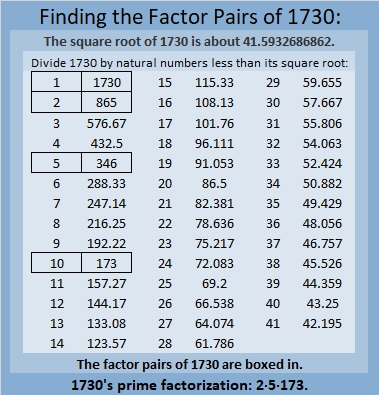# How Is 1730 the Sum of Consecutive Squares?

Contents

### Today’s Puzzle:

Write all the numbers from 1 to 12 in both the first column and the top row so that those numbers are the factors of the given clues.### Factors of 1730:

• 1730 is a composite number.
• Prime factorization: 1730 = 2 × 5 × 173.
• 1730 has no exponents greater than 1 in its prime factorization, so √1730 cannot be simplified.
• The exponents in the prime factorization are 1, 1, and 1. Adding one to each exponent and multiplying we get (1 + 1)(1 + 1)(1 + 1) = 2 × 2 × 2 = 8. Therefore 1730 has exactly 8 factors.
• The factors of 1730 are outlined with their factor pair partners in the graphic below.### More about the number 1730:

1730 is the sum of two squares in two different ways:
41² + 7² = 1730, and
37² + 19² = 1730.

1730 is the hypotenuse of FOUR Pythagorean triples:
520 1650 1730 which is 10 times (52-165-173)
574 1632 1730 calculated from 2(41)( 7), 41² – 7², 41² + 7²,
1008 1406 1730 calculated from 37² – 19², 2(37)(19), 37² + 19², and
1038 1384 1730 which is 346 times (3-4-5).

Finally, OEIS.org informs us that 1730 is the sum of consecutive squares in two different ways. What are those two ways? I figured it out. Can you?

Here’s a hint: It is the sum of three consecutive squares as well as twelve consecutive squares. That means √(1730/3) rounded is included in one sum and √(1730/12) rounded is included in the other. The solution can be found in the comments. Have fun finding them yourself though!

## One thought on “How Is 1730 the Sum of Consecutive Squares?”

1.ivasallay

23² + 24² + 25² = 1730, and
6² + 7² + 8² + 9² + 10² + 11² + 12² + 13² + 14² + 15² + 16² + 17² = 1730.

This site uses Akismet to reduce spam. Learn how your comment data is processed.